Products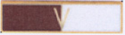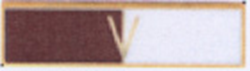### A12701

(image does not represent actual size)
Dimensions: 1 3/8" (W) x 3/8" (H)
A12701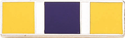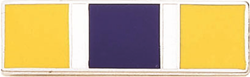### A1475

(image does not represent actual size)
Dimensions: 1 3/8" (W) x 3/8" (H)
A1475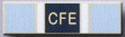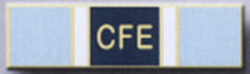### A1475-C

(image does not represent actual size)
Dimensions: 1 3/8" (W) x 3/8" (H)
A1475-C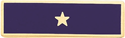### A346

(image does not represent actual size)
Dimensions: 1 7/16" (W) x 7/16" (H)
A346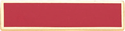### A4616-A

(image does not represent actual size)
Dimensions: 1 3/8" (W) x 5/16" (H)
A4616-A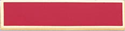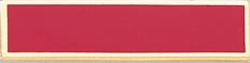### A4616-ALS

(image does not represent actual size)
Dimensions: 1 3/8" (W) x 5/16" (H)
A4616-ALS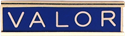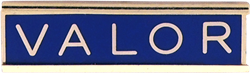### A4616-AX

(image does not represent actual size)
Dimensions: 1 3/8" (W) x 5/16" (H)
A4616-AX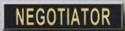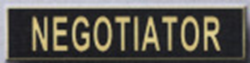### A4616-AY

(image does not represent actual size)
Dimensions: 1 3/8" (W) x 5/16" (H)
A4616-AY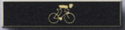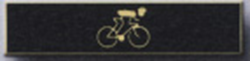### A4616-AZ

(image does not represent actual size)
Dimensions: 1 3/8" (W) x 5/16" (H)
A4616-AZ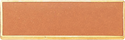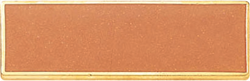### A7140

(image does not represent actual size)
Dimensions: 1 3/8" (W) x 3/8" (H)
A7140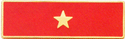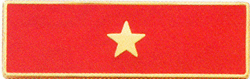### A7140-C

(image does not represent actual size)
Dimensions: 1 3/8" (W) x 3/8" (H)
A7140-C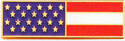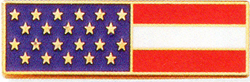### A7140-J

(image does not represent actual size)
Dimensions: 1 3/8" (W) x 3/8" (H)
A7140-J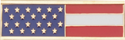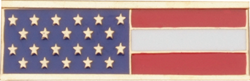### A7140-JLS

(image does not represent actual size)
Dimensions: 1 3/8" (W) x 3/8" (H)
A7140-JLS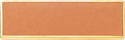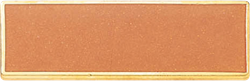### A7140-LS

(image does not represent actual size)
Dimensions: 1 3/8" (W) x 3/8" (H)
A7140-LS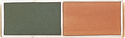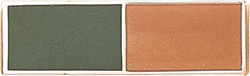### A7141

(image does not represent actual size)
Dimensions: 1 3/8" (W) x 3/8" (H)
A7141